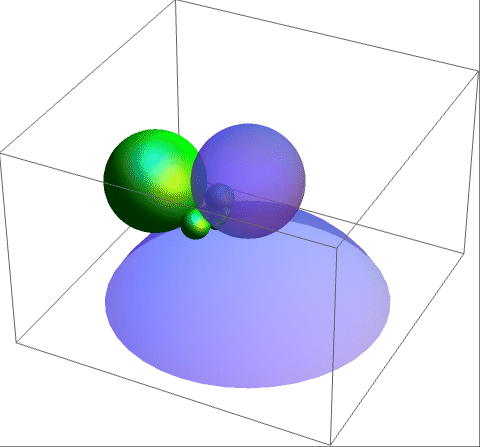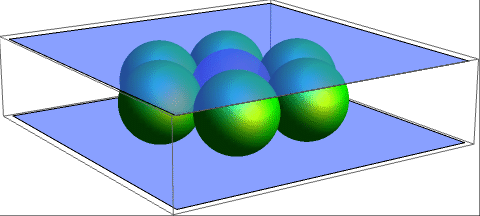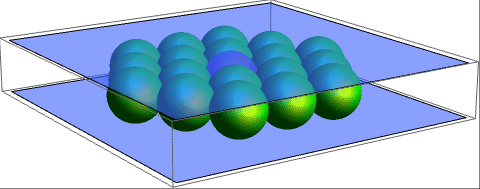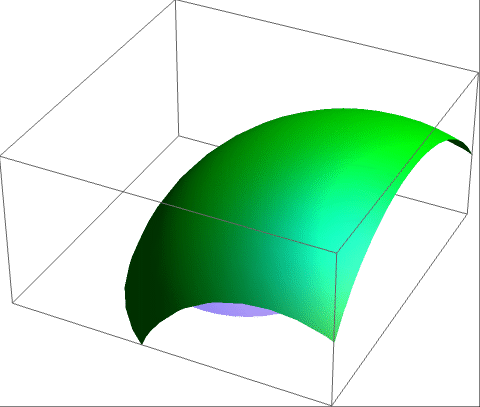# Soddy’s hexlet

Suppose we have three semi-transparent light blue spheres, S1, S2 and S3, which are all externally tangent to each other. Then, we have a series of metallic green spheres, Γ0, Γ1, Γ2, Γ3, Γ4, Γ5, such that each one touches the previous one and the three light blue spheres. It is a remarkable fact that they complete a chain (Γ5 touches Γ0), irrespective of the original three light blue spheres. Of course, there is a one-parameter family of possible starting locations for Γ0, as visualised below:There is quite a simple explanation for this fact. Recall that we can append a point at infinity to$\mathbb{R}^3$ to obtain a topological 3-sphere, and we can give an exact mapping to the unit 3-sphere in 4-space by means of stereographic projection. Then, the family of projective transformations of 4-space which fix the unit 3-sphere map planes to planes, and therefore 2-spheres on the unit 3-sphere (intersections of planes with the unit 3-sphere) to 2-spheres.

In other words, we obtain a group of conformal transformations mapping spheres to spheres (or planes). Now, if we send the tangency point of two of the blue spheres to infinity, those two blue spheres will become parallel planes sandwiching a third blue sphere, as in the following situation:But all spheres that are mutually tangent to both planes must necessarily have the same radius, and taking a cross-section halfway between the two planes reduces this to a very simple problem: how many unit-radius circles can simultaneously touch another unit-radius circle? The answer, of course, is six, as epitomised by the hexagonal packing of circles.

Suddenly, at this very moment, I had a realisation: we can add extra layers of spheres!And reapply the transformation of (real!) projective 4-space to give a less trivial example:Note that for any orbit of metallic green spheres, the envelope is a transformed torus, or Dupin cyclide. The orbit can be classified as elliptic (if the envelope is bounded), parabolic (if it touches the point at infinity), or hyperbolic (if the envelope contains the point at infinity). Now, when we have more than six spheres (as in the example above), we have multiple orbits, which may be of different types.

The spheres on parabolic orbits grow infinite in size before shrinking again. The hyperbolic orbits are even worse, with spheres temporarily turning inside-out as they engulf the point at infinity! The following animation shows all three types in some crazy uncivilised menage-a-trois:This entry was posted in Uncategorized. Bookmark the permalink.

### One Response to Soddy’s hexlet

1.roman33310 says:

I have a problem with the annular hexlet. The question is the friction between the spheres. If the center sphere dictates the rotation then the outer six spheres, and those spheres act against one another, thus a point of contact, how are they allowed to rotate given this observation?